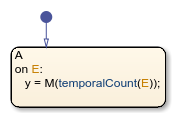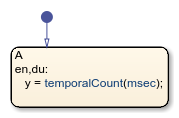# temporalCount

Number of events, chart executions, or time since state became active

## Syntax

``temporalCount(E)``
``temporalCount(tick)``
``temporalCount(time_unit)``

## Description

example

````temporalCount(E)` returns the number of occurrences of the event `E` since the associated state became active.```

example

````temporalCount(tick)` returns the number of times that the chart has woken up since the associated state became active.The implicit event `tick` is not supported when a Stateflow® chart in a Simulink® model has input events.```

example

````temporalCount(time_unit)` returns the length of time that has elapsed since the associated state became active. Specify `time_unit` as seconds (`sec`), milliseconds (`msec`), or microseconds (`usec`).```

Note

Standalone Stateflow charts in MATLAB® support using `temporalCount` only as an absolute-time temporal logic operator.

## Examples

expand all

Access successive elements of the array `M` each time that the chart processes a broadcast of the event `E`.

In charts in a Simulink model, enter:

``````on E: y = M(temporalCount(E));``````Using `temporalCount` as an event-based temporal logic operator is not supported in standalone charts in MATLAB.

Store the value of the input data `u` in successive elements of the array `M`.

In charts in a Simulink model, enter:

```en,du: M(temporalCount(tick)+1) = u;```Using `temporalCount` as an event-based temporal logic operator is not supported in standalone charts in MATLAB.

Store the number of milliseconds since the state became active.

```en,du: y = temporalCount(msec);```## Tips

• You can use quotation marks to enclose the keywords `'tick'`, `'sec'`, `'msec'`, and `'usec'`. For example, `temporalCount('tick')` is equivalent to `temporalCount(tick)`.

• The Stateflow chart resets the counter used by the `temporalCount` operator each time the associated state reactivates.

• The timing for absolute-time temporal logic operators depends on the type of Stateflow chart:

• Charts in a Simulink model define temporal logic in terms of simulation time.

• Standalone charts in MATLAB define temporal logic in terms of wall-clock time.

The difference in timing can affect the behavior of a chart. For example, suppose that this chart is executing the `entry` action of state `A`.• In a Simulink model, the function call to `f` executes in a single time step and does not contribute to the simulation time. After calling the function `f`, the chart assigns a value of zero to `y`.

• In a standalone chart, the function call to `f` can take several seconds of wall-clock time to complete. After calling the function `f`, the chart assigns the nonzero time that has elapsed since state `A` became active to `y`.

## Version History

Introduced in R2008a